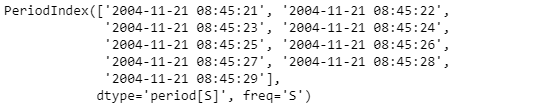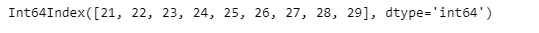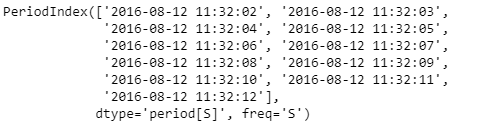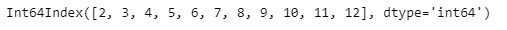Skip to content
Related Articles
Python | Pandas PeriodIndex.second
• Last Updated : 06 Jan, 2019

Python is a great language for doing data analysis, primarily because of the fantastic ecosystem of data-centric python packages. Pandas is one of those packages and makes importing and analyzing data much easier.

Pandas` PeriodIndex.second` attribute return an Index object containing the second values for each period element present in the given PeriodIndex object.

Syntax : PeriodIndex.second

Parameters : None

Return : Index object

Example #1: Use `PeriodIndex.second` attribute to find out the second values present in each period element in the given PeriodIndex object.

 `# importing pandas as pd``import` `pandas as pd`` ` `# Create the PeriodIndex object``pidx ``=` `pd.PeriodIndex(start ``=``'2004-11-21 08:45:21 '``, ``               ``end ``=``'2004-11-21 8:45:29'``, freq ``=``'S'``)`` ` `# Print the PeriodIndex object``print``(pidx)`

Output :Now we will use the `PeriodIndex.second` attribute to find out the second values for each period element in pidx object.

 `# return the second values``pidx.second`

Output :As we can see in the output, the `PeriodIndex.second` attribute has returned seconds object containing the seconds value for each period in the given PeriodIndex object.

Example #2: Use `PeriodIndex.second` attribute to find out the second values present in each period element in the given PeriodIndex object.

 `# importing pandas as pd``import` `pandas as pd`` ` `# Create the PeriodIndex object``pidx ``=` `pd.PeriodIndex(start ``=``'2016-8-12 11:32:02'``, ``            ``end ``=``'2016-08-12 11:32:12'``, freq ``=``'S'``)`` ` `# Print the PeriodIndex object``print``(pidx)`

Output :Now we will use the `PeriodIndex.second` attribute to find out the second values for each period element in pidx object.

 `# return the second values``pidx.second`

Output :As we can see in the output, the `PeriodIndex.second` attribute has returned an Index object containing the seconds value for each period in the given PeriodIndex object.

Attention geek! Strengthen your foundations with the Python Programming Foundation Course and learn the basics.

To begin with, your interview preparations Enhance your Data Structures concepts with the Python DS Course. And to begin with your Machine Learning Journey, join the Machine Learning – Basic Level Course

My Personal Notes arrow_drop_up